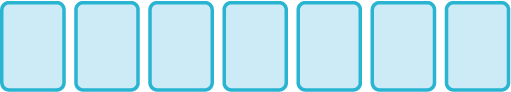#### You may also like### Consecutive Numbers

An investigation involving adding and subtracting sets of consecutive numbers. Lots to find out, lots to explore.### Roll These Dice

Roll two red dice and a green dice. Add the two numbers on the red dice and take away the number on the green. What are all the different possible answers?### Domino Square

Use the 'double-3 down' dominoes to make a square so that each side has eight dots.

# Fifteen Cards

##### Age 7 to 11Challenge Level

I have fifteen cards numbered $1 - 15$.

I put down seven of them on the table in a row.The numbers on the first two cards add to $15$.
The numbers on the second and third cards add to $20$.
The numbers on the third and fourth cards add to $23$.
The numbers on the fourth and fifth cards add to $16$.
The numbers on the fifth and sixth cards add to $18$.
The numbers on the sixth and seventh cards add to $21$.

What are my cards?

Can you find any other solutions?

How do you know you've found all the different solutions?